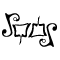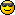# Math Is Fun Forum

Discussion about math, puzzles, games and fun.   Useful symbols: ÷ × ½ √ ∞ ≠ ≤ ≥ ≈ ⇒ ± ∈ Δ θ ∴ ∑ ∫  π  -¹ ² ³ °

You are not logged in.

## #1 2007-03-09 17:05:37

tigerfan
Member
Registered: 2007-03-09
Posts: 8

the problem is i'm lost

a arrow is fired with a velocity of 45 m/s at an angle of 50 degrees.  what is the height of the arrow 150 meters away.

Offline

## #2 2007-03-09 18:36:29

Stanley_Marsh
MemberRegistered: 2006-12-13
Posts: 345

Give you a tip , you can divide the v into two v on x-axis and y-axis.
the v on y-axis describe the motion in height , and the x-axis in lenght.  Two motion are independent.

Numbers are the essence of the Universe

Offline

## #3 2007-03-09 19:20:49

ryos
MemberRegistered: 2005-08-04
Posts: 394

...and to do that, you treat the velocity as a vector quantity of magnitude 45 and making an angle of 50 degrees with the x-axis. You can then find the individual components as the legs of a right triangle, like so:

v = 45 * <sin(50), cos(50)>

I've bolded v to denote that it's a vector quantity, and the angle brackets (<>) denote a vector with the x portion first and the y portion second. The 45 distributes through to both.

This is your initial velocity. Because we're ignoring air resistance (you are usually allowed in these problems since modeling air resistance can get complicated), the x component does not change for the entire time. This lets us find the amount of time it will take the arrow to reach 150 meters:

t = 150m / (45*sin(50) (m/s))

This gives the time in seconds. We now know everything necessary to apply the formula for the position of an object under constant acceleration to find the height of the arrow.

The formula is: s = x0 + v0*t + (1/2)a*t²
...where s means "position" for some reason, and "x0" and "v0" are the initial position and velocity. Though I've given the vector form of the formula, in this problem we are only concerned with one dimension so we can use the scalar form. Now:
x0 = 0
v0 = 45 * cos(50)
t = 150 / (45 * sin(50))
a = -g = -9.8 m/s²

Plug those into the formula, and you'll have your answer.El que pega primero pega dos veces.

Offline